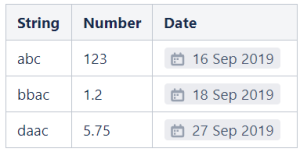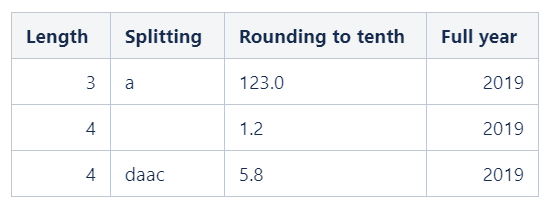Page tree
Go to start of banner

List of SQL functions available

Сolumn and constant syntax

DefinitionCharacterExample
Column nameSingle quotes'Column name'
String constantDouble quotes

"Some constant"

"Free text"

"Date"

Functions and keywords

Oops, it seems that you need to place a table or a macro generating a table within the Table Filter macro.

Function / KeywordTypeDescription
ABS

MATH

Returns the absolute (positive) value of a number

MATH

TIME

Adds time values (as intervals) to a date value
ALL

LOGIC

Returns true if all of the subquery values meet the condition
AND

LOGIC

Includes rows where all the conditions separated by AND are TRUE
ANY

LOGIC

Returns true if any of the subquery values meet the condition
ARRAY

DATA_MANIPULATING

Creates an array
AS

DATA_MANIPULATING

Renames a column or table with an alias
ASC

MATH

ORDERING

Ascending sorting order
AT

TIME

AVG

MATH

AGGREGATION

Returns the average value of a group
BEGIN

LOGIC

LOOP

Starts code block for WHILE and IF statements
BETWEEN

MATH

Selects values within a given range
BY

AGGREGATION

ORDERING

Used with ORDER BY, GROUP BY
CASE

DATA_MANIPULATING

LOGIC

Creates different outputs based on conditions (see an example)
CAST

DATA_MANIPULATING

CONVERTING

Converts a value (of any type) into a specified datatype
CEIL

MATH

Gets the smallest integer which is greater than, or equal to, the specified numeric expression
CHAR

STRING_OPERATORS

Returns the character with the specified integer unicode
CHECK

LOGIC

Limits the value that can be placed in a column
COALESCE

LOGIC

Returns the first non-null value in a list
CORRESPONDING

MERGING

Matches the columns in table-expressions by name and not by ordinal position
COUNT

MATH

AGGREGATION

Returns the number of rows that matches a specified criteria
CONCAT

MERGING

Concatenates values
CROSS

MERGING

One of the Join types. Returns all rows for all possible combinations of two tables
CUBE

MATH

AGGREGATION

Generates subtotals for all combinations of grouping columns specified in the GROUP BY clause
CURRENT_TIMESTAMP

VARIABLES

TIME

Returns the current timestamp of the operating system of the server on which the SQL Server Database runs
DATE

DATA_MANIPULATING

CONVERTING

A data type for storing a date (string like 'YYYYMMDD')

MATH

TIME

Adds time values (as intervals) to a date value

MATH

TIME

Adds time values (as intervals) to a date value

MATH

TIME

Adds time values (as intervals) to a date value
DATE_SUB

MATH

TIME

Subtracts a time/date interval from a date and then returns the date
DATEDIFF

MATH

TIME

Returns the difference between two dates
DAY

DATA_MANIPULATING

TIME

CONVERTING

Returns the day of the month for a date
DEFAULT

DATA_MANIPULATING

LOGIC

Provides a default value for a column
DESC

MATH

ORDERING

Descending sorting order
DISTINCT

LOGIC

AGGREGATION

Selects only distinct (different) values
ELSE

LOGIC

Introduces another statement that is executed when the IF condition is not satisfied
END

LOGIC

Completes code block for WHILE and IF statements
ESCAPE

LOGIC

SEARCHING

Equals to LIKE
ESCAPEMARKUP

STRING_OPERATORS

Escapes special characters for wiki markup
EXCEPT

MERGING

Combine two SELECT statements and returns rows from the first SELECT statement that are not returned by the second SELECT statement
EXISTS

LOGIC

Tests for the existence of any record in a subquery
EXP

MATH

Returns e raised to the power of the specified number
FIRST

AGGREGATION

Returns the first value in the group
FLOOR

MATH

Returns the largest integer value that is smaller than or equal to a number
FORMATDATE

DATA_MANIPULATING

TIME

Converts dates to the specified in the Table Transformer macro settings date format: FORMATDATE (calculated_date)
FORMATMARKDOWN

FORMATTING

Applies Markdown syntax to table cells
FORMATNUMBER

DATA_MANIPULATING

FORMATTING

Format numbers according to the settings in the Table Transformer macro: FORMATNUMBER (calculated_number) .
FORMATTIME

DATA_MANIPULATING

TIME

Converts time to the specified in the Table Transformer macro settings time format to the calculated time: FORMATTIME (calculated_time)
FORMATWIKI

FORMATTING

Applies Confluence Wiki Markup to table cells
FORMATWORKLOG

DATA_MANIPULATING

TIME

Converts worklogs to the specified in the Table Transformer macro worklog settings: FORMATWORKLOG (calculated_worklog)
FROM

KEYWORD

Specifies which table to select or delete data from
FULL

MERGING

FULL OUTER JOIN: returns all rows when there is a match in either left table or right table
GREATEST

MATH

AGGREGATION

Returns the greatest value of the list of arguments
GROUP

AGGREGATION

Groups the result set (used with aggregate functions: COUNT, MAX, MIN, SUM, AVG)
GROUPING

LOGIC

AGGREGATION

Indicates whether the specified column in a GROUP BY Clause aggregated or not
HAVING

LOGIC

AGGREGATION

Used instead of WHERE with aggregate functions
HOUR

DATA_MANIPULATING

TIME

Returns the hour part of a datetime
IF

LOGIC

Conditional statement
IFNULL

LOGIC

Returns a specified value if the expression is NULL
IIF

LOGIC

Accepts three arguments. It evaluates the first argument and returns the second argument if the first argument is true; otherwise, it returns the second argument
IN

LOGIC

Allows you to specify multiple values in a WHERE clause.

INNER

MERGING

INNER JOIN: returns rows that have matching values in both tables
INSTR

STRING_OPERATORS

Returns the position of the first occurrence of a string in another string
INTERSECT

MERGING

Intersects two SELECT results
INTERVAL

TIME

Defines a date/time interval
IS

LOGIC

ISNULL

LOGIC

Returns TRUE or FALSE depending on whether an expression is NULL

JOIN

MERGING

Joins tables (see an example)
LCASE

STRING_OPERATORS

Converts a string to lower-case
LEAST

MATH

AGGREGATION

Returns the smallest value of the list of arguments
LEFT

MERGING

LEFT JOIN: returns all rows from the left table, and the matching rows from the right table
LENGTH

STRING_OPERATORS

Returns the length of a string (in bytes)
LIKE

LOGIC

SEARCHING

Searches for a specified pattern in a column
LIMIT

SEARCHING

Limit the number of records from the result set
LOG

MATH

Returns the natural logarithm
MAX

MATH

AGGREGATION

Takes the name of a column as an argument and returns the largest value in that column
MEDIAN

MATH

AGGREGATION

Returns the median value of a group (see an example)
MID

STRING_OPERATORS

Extracts a substring from a string (starting at any position)
MIN

MATH

AGGREGATION

Takes the name of a column as an argument and returns the smallest value in that column
MINUTE

DATA_MANIPULATING

TIME

Returns the minute part of a datetime value
MONTH

DATA_MANIPULATING

TIME

Returns the month part of a date
MULT

MATH

AGGREGATION

Takes the name of a column as an argument and returns multiplying of all the values in that column

NATURAL

MERGING

NATURAL JOIN: analyzes columns in two joined tables and makes a join by columns with similar names
NOT

LOGIC

Includes rows where a condition is not TRUE
NOW

VARIABLES

Returns current date and time
NULL

LOGIC

A field with no value
NULLIF

LOGIC

Compares two expressions and returns NULL if they are equal. Otherwise, the first expression is returned
OF

LOGIC

Allows you to specify multiple values in a WHERE clause
OFFSET

SEARCHING

Skips the first N rows in a result set before starting to return any rows
ON

MERGING

OR

LOGIC

Includes rows where either condition is TRUE
ORDER

ORDERING

Sorts the result set in ascending or descending order
OUT

LOGIC

Opposite to IN
OUTER

MERGING

OUTER JOIN: returns all rows when there is a match in either left table or right table
PERCENT

SEARCHING

Indicates that the query returns N percentage of rows
POWER

MATH

Returns the value of a number raised to the power of another number
QUART

MATH

AGGREGATION

Returns the first quartile of a group
QUART2

MATH

AGGREGATION

Returns the second quartile of a group
QUART3

MATH

AGGREGATION

Returns the third quartile of a group
RANDOM

VARIABLES

REGEXP_LIKE

LOGIC

STRING_OPERATORS

SEARCHING

Returns rows that match a regular expression pattern
REPLACE

STRING_OPERATORS

Replaces all occurrences of a substring within a string, with a new substring
RIGHT

MERGING

RIGHT JOIN: returns all rows from the right table, and the matching rows from the left table
ROLLUP

MATH

AGGREGATION

Creates subtotals and grand totals for a set of columns (GROUP BY ROLLUP)
ROUND

MATH

Rounds a number to a specified number of decimal places
ROWNUM

DATA_MANIPULATING

Adds row numbering to a table
SECOND

DATA_MANIPULATING

TIME

Return the seconds part of a datetime value
SELECT

DATA_MANIPULATING

Selects data from a database
SOME

LOGIC

Compares a value to each value in a list or results from a query and evaluates to TRUE if the result of an inner query contains at least one row
SQRT

MATH

Returns the square root of a number
STDDEV

MATH

AGGREGATION

Returns the population standard deviation of expression (see an example)
STDEV

MATH

AGGREGATION

Returns the sample standard deviation of expression (see an example)
SUBDATE

MATH

TIME

Subtracts a time/date interval from a date and then returns the date
SUBSTR

STRING_OPERATORS

Extracts a substring from a string (starting at any position)
SUBSTRING

STRING_OPERATORS

Allows to extract a substring from a string
SUM

MATH

AGGREGATION

Takes the name of a column as an argument and returns the sum of all the values in that column (see an example)
THEN

LOGIC

A result expression in a search condition
TIME

DATA_MANIPULATING

TIME

Extracts the time part from a given time/datetime

CONVERTING

Returns a datetime value based on a date or datetime value

TO

KEYWORD

STRING_OPERATORS

Removes the space character or other specified characters from the start or end of a string
UCASE

STRING_OPERATORS

Converts a string to upper-case
UNION

MERGING

Combines the result set of two or more SELECT statements (only distinct values)
UPPER

STRING_OPERATORS

Converts a string to upper-case
USING

LOGIC

Matches only one column when more than one column matches
VAR

MATH

AGGREGATION

Returns the statistical variance of all values in the specified expression
VAR_POP

AGGREGATION

Population variance. Returns 0 if all of the values in the data set have the same value (no variability). Returns 0 if the data set consists of only one value (no possible variability). Returns NULL if the data set has no values
VAR_SAMP

AGGREGATION

Sample variance. Returns 0 if all of the values in the data set have the same value (no variability). Returns NULL if the data set consists of only one value (no possible variability). Returns NULL if the data set has no values
VARCHAR

KEYWORD

Accepts character strings of a variable length is up to the maximum length specified in the data type declaration
VARP

AGGREGATION

Returns the Variance for the population of the total number of records present in the specified column
WEEKDAY

DATA_MANIPULATING

TIME

Returns the weekday number for a given date
WHEN

LOGIC

A condition expression in a search condition
WHERE

LOGIC

SEARCHING

Filters a result set to include only records that fulfill a specified condition
WHILE

LOOP

Sets a condition for the repeated execution of an SQL statement or statement block
YEAR

DATA_MANIPULATING

TIME

Returns an integer value which represents the year of the specified date
FALSE

LOGIC

Logical false value
TRUE

LOGIC

Logical true value

• ::Date
• ::string
• ::number

JavaScript methods

After converting to date you can use JavaScript date functions, for example:

'Column with dates'::Date→toDateString()

Find an example here.

After converting to string you can use JavaScript string functions, for example:

'Column with strings'::string->split("The delimiter")->0

'Column with strings'::string→length

After converting to number you can use JavaScript number functions, for example:

'Column with numbers'::number→toFixed(1)

You need to convert only values with a different or indefinite type. In other cases, it's is not necessary:

'Column with numbers'->toFixed(1)

Example

The source table:The resultSQL query:

SELECT
T1.'String'->length AS 'Length',
T1.'String'->split("b")->0 AS 'Splitting',
T1.'Number'->toFixed(1) AS 'Rounding to tenth',
T1.'Date'::Date->getFullYear() AS 'Full year'
FROM T*